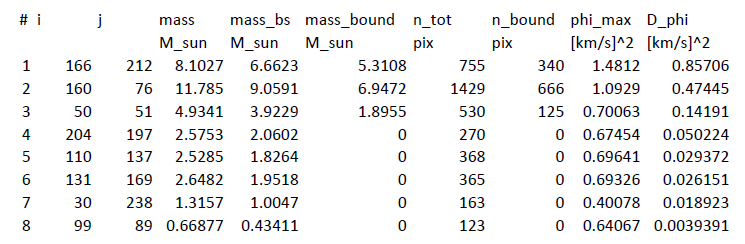Gravitational Potential Identification of Cores

GRID-core is a core-finding method using the contours of the local gravitational potential

to identify core boundaries, as described in Gong & Ostriker (2011). There it is shown that the

GRID-core method applied to 2D surface density and 3D volume density are in good agreement,

for bound cores.

We have implemented a version of the GRID-core algorithm in IDL, suitable for core-finding in

observed maps. The required input is a two-dimensional FITS file containing a map of the column

density in a region of a cloud.

The user's guide is available in PDF format.

( Gong & Ostriker 2011) if you use the code for presentation or publication.

2. Gravitational potential of surface density

3. The largest closed contour

4. Bound thermal cores

5. Structure of the IDL progam

6. Running the IDL program

7. Testing the code

1. Introduction

GRID-core (Gravitational potential Identification of cores) is a core-finding method using the
contours of the local gravitational potential to identify core boundaries, as described in Gong &
Ostriker (2011)
. There it is shown that the GRID-core method applied to 2D surface density and 3D
volume density are in good agreement, for bound cores. This user guide describes how to implement
this method on observed surface density. We describe the algorithms used to find the largest closed
contour that defines the outer core limit, and to identify the gravitationally bound interior part of
the core. In addition, we describe use of the IDL code to implement GRID core-finding on FITS
maps.

2. Gravitational potential of surface density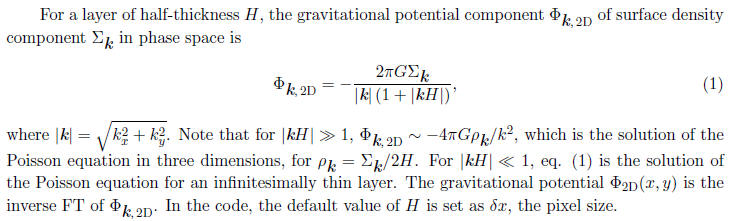3. The largest closed contour

To identify cores via the gravitational potential, we first find and mark all the local minima
of the gravitational potential; second, we find the largest closed potential contour surrounding
each individual minimum. In the second step, we increase the contour level from the bottom of a
given potential well step by step until it violates another minimum's marked territory by enclosing
more than one extremum of the potential. We demonstrate this procedure in Figure 1 using a
one dimensional positive potential, -Φ . In the first step, local maxima (minima in Φ) are marked
in a descending order; note that only pixels at extrema are marked in this step. For the second
step, we take the local maximum "1" as an example. Starting at | Φmin|, we decrease the isopotential
contour level by amount of ΔΦ from the top of "1", and find the region which is connected to "1" for
each contour level. The blue lines in Figure 1 show the contour levels, and the dotted blue lines show
the identified connected regions to the maximum "1". At the 7th contour level, the connected region
violates the territories of other maxima, because more than one extremum is contained within the
contour (i.e. lying above the lowest blue line, in Fig.1) . The largest closed contour of "1" is
thus defined by the 6th contour level, marked by the red lines. We repeat this procedure on all the
local maxima and mark the largest closed contour of each maximum.

The contour interval ΔΦ has a negligible effect on the results as long as it is small enough. We
define the region enclosed by the largest closed contour as a GRID-core. If the distance between
two potential minima is smaller than a certain value of pixels (corresponding to a physical distance
which is set by the resolution), the regions associated with these two minima are merged and treated
as a single GRID-core.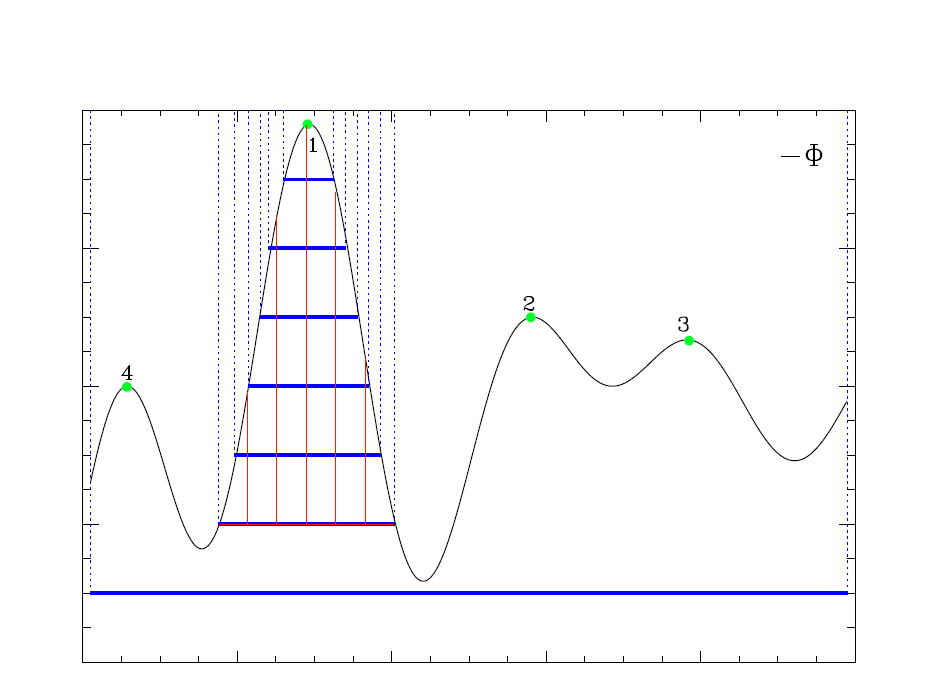Fig. 1. Schematic of GRID-core identification method.

4. Bound thermal cores

Gas with sufficient thermal (and kinetic) energy will not be permanently (or even temporarily)
bound to a given core, so the gravitational potential is not the final word. The lower density outer
parts of a core are the least bound, and most subject to mass loss.

In order to identify only the bound regions of cores as marked by the gravitational potential,
we add thermal energy to the gravitational energy, and only assign a given fluid element to a
core if Eth+Eg < 0. For any fluid element, the specific thermal energy is taken to be Eth = (3/2)cs2,
for cs the isothermal sound speed, and the specific gravitational potential energy is taken to be
Egmin - Φmax, where Φmax is the potential of the largest closed contour that defines the core. In
the example of section 2, Φmax would be equal to the potential of the sixth contour, i.e. -Φ max =
- Φmin - 6Δ Phi. Including a thermal energy condition decreases the area so that (instantaneously)
bound cores are smaller than cores defined by the potential alone. The resulting region is defined
as a bound GRID-core.

Of course, the thermal energy can in fact be radiated away, so that gas that is initially near
the largest closed contour may become more strongly bound after the interior of a core collapses.
Thus, the gas within the outer (unbound) GRID-core region could evolve to become a bound core
eventually.

Since bound cores must have Φmax −Φmin < (3/2)cs2, a value ΔΦ ~ 0.1cs2 is typically suitable
for the potential contour spacing in identifying core boundaries. This is the default value adopted
in the code.

We define a background surface density as the mean of the bottom 10% of the surface density;
this mean value can be subtracted from the surface density in the core region when calculating core
masses.

5. Structure of the IDL progam

eliminates unresolved cores (total pixel number smaller than π r_pix_lim2). The subroutine
"boundcore2d" calculates core properties such as the coordinates of core center, total mass of the region
inside the largest closed contour, mass of the bound core region, the depth of the gravitational
potential well ( Φmax −Φmin), and the total number of pixel numbers in marked cores, and bound
regions. All the other procedures such as calculating the gravitational potential, and core-finding
are handled in the main function. This section explains each block in the main function.
The first block is to calculate the gravitational potential of surface density. The surface density
in g cm−2 is obtained by multiplying input H column density by 1.42mp. To create a periodic input
for the FFT function, we zero-pad the surface density map in a domain four times as large, putting
the physical surface density in the lower left quarter and zeros in the other three quarters. The next step is
to apply a forward FFT to the extended surface density map. After multiplying by the coefficients
as in equation (1), we apply a backward FFT to obtain the gravitational potential of the extended
surface map. At the end of this block, the bottom left part of the gravitational potential field is
extracted for later core-finding. Notice that the potential field is converted to positive values.
The second block is to merge local maxima (originally these are minima, since we use −Φ
instead of Φ) if they are too close to each other (rdistance < cls_dis).
The third block is to do GRID core-finding. The algorithm is described in Section 3. The
block to eliminate unresolved cores is right after this block.

6. Running the IDL program

The calling sequence is:

output=grid core(filename, pix_size,T,cls_dist=cls_dist,dp=dp,h=h,r_pix lim=r_pix_lim)

ARGUMENTS:

filename : the name of the FITS ﬁle containing column density, where the maps is assumed to

represent NH of H nuclei (not H2), in units of cm-2

pix_size : the pixel resolution of the input column density map, in units of pc

KEYWORDS:

T             : temperature of the cloud (assumed isothermal), in units of K; the default value is 10 K.

dp           : the interval between potential contour levels, in units of cs2 = kT /µ; the default value is 0.1.

cls_dist   : the closest distance between two local potential minima, in units of pix size; the default value is 6.

h             : the minimum thickness of the 2D layer, in units of pix_size; the default value is 1.

r_pix_lim : the minimum radius for a core to be considered resolved, in units of pix size; the default value is 3,

half of cls_dist.

OUTPUTS:

1. Two FITS files containing the marked regions identifying cores, e.g.: lcc_0.100.fits (GRID- cores),

lcc_b_0.100.fits (bound GRID-cores). The suffix gives the value of dp (“0.100” for the default).

2.  A FITS file containing a map of the computed gravitational potential phi.fits ( in units of [km/s]2).

3.  A data file containing the properties of each core: location of potential minimum, total mass of marked region,

background-subtracted mass of bound region, pixel numbers of both total and bound regions, gravitational

potential |Φmin| at the core center, gravitational potential depth Φmax −Φmin.

4. A postscript file showing the marked regions identifying cores: core_on_surfd_0.100.ps .

Examples of how to run the code:

1. Setting all keywords –

.run grid_core

output=grid_core(“column.fits”,0.011,10.,cls dist=6,dp=0.01,h=1.0,r pix_lim=3)

2.Using default keywords –

.run grid_core

output=grid_core(“column.fits”,0.011,10.)

7. Testing the code

For a column density map (figure 2, 256x256), the potential map is shown in Fig. 3, and the marked GRID-core

and bound GRID-core areas are shown in Fig. 4 and Fig. 5 respectively. Fig. 6 shows the marked GRID-cores

and bound GRID-cores on the column density map.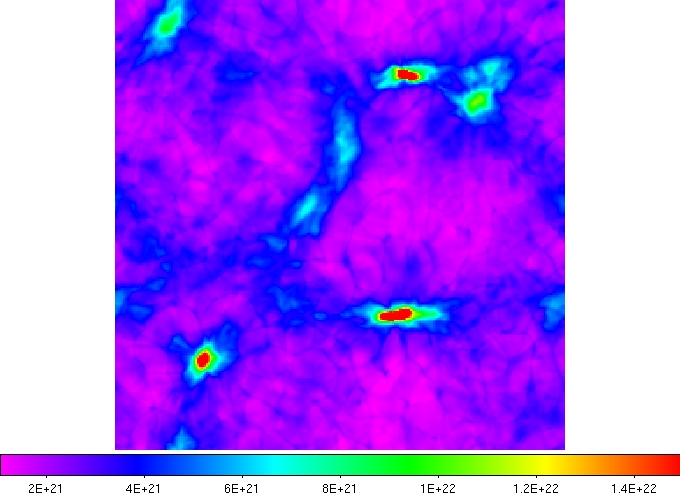Fig. 2  Column density map. Color scale represents the column density (logNH).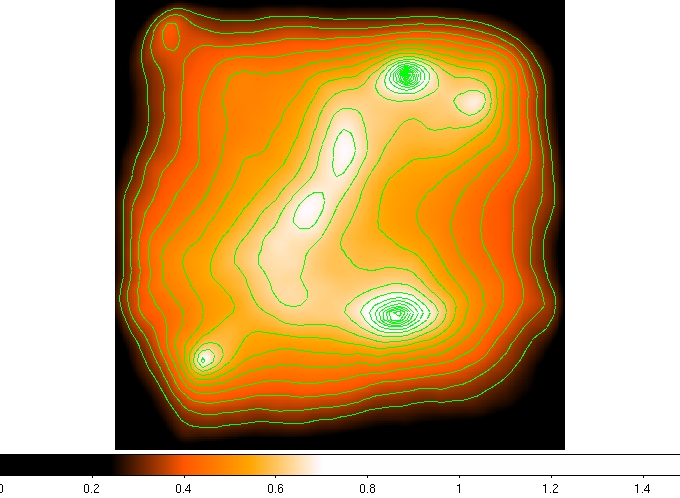Fig. 3  Potential map of the above column density map. Green curves show the contours.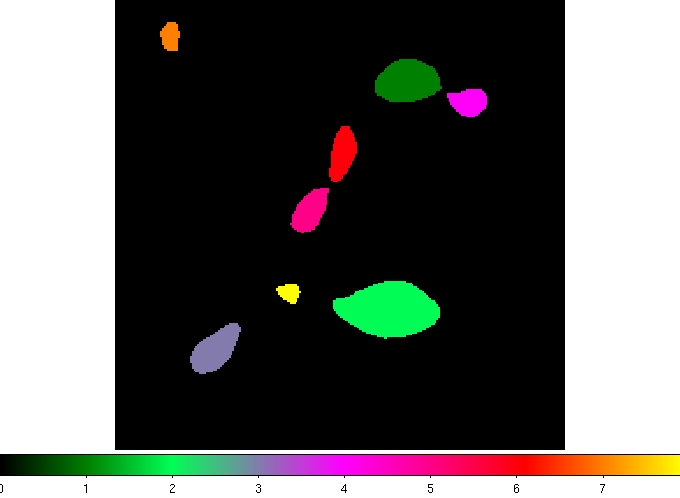Fig. 4  Identified GRID-cores. Colors represent different core indices.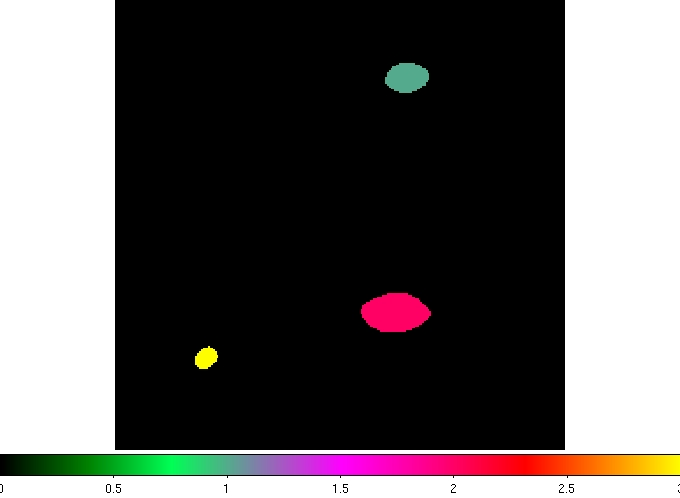Fig. 5  Identified bound GRID-cores.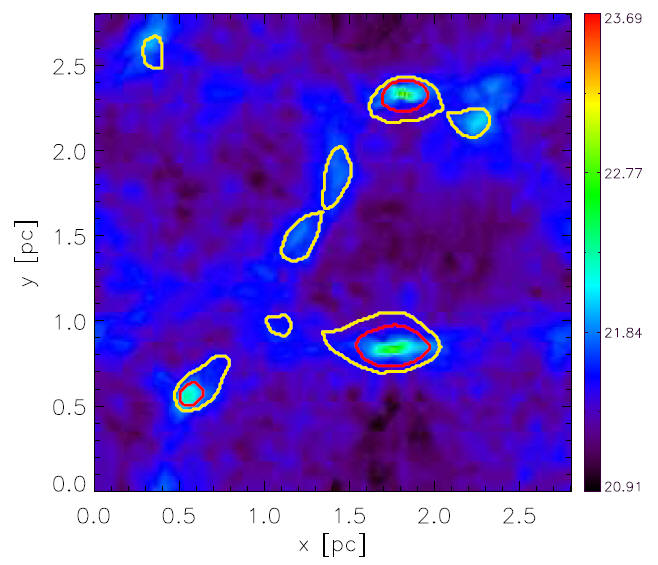Fig. 6  Identified GRID-cores (yellow curves) and bound GRID-cores (red curves) superposed on the

column density.

The parameters of identified cores are list below: